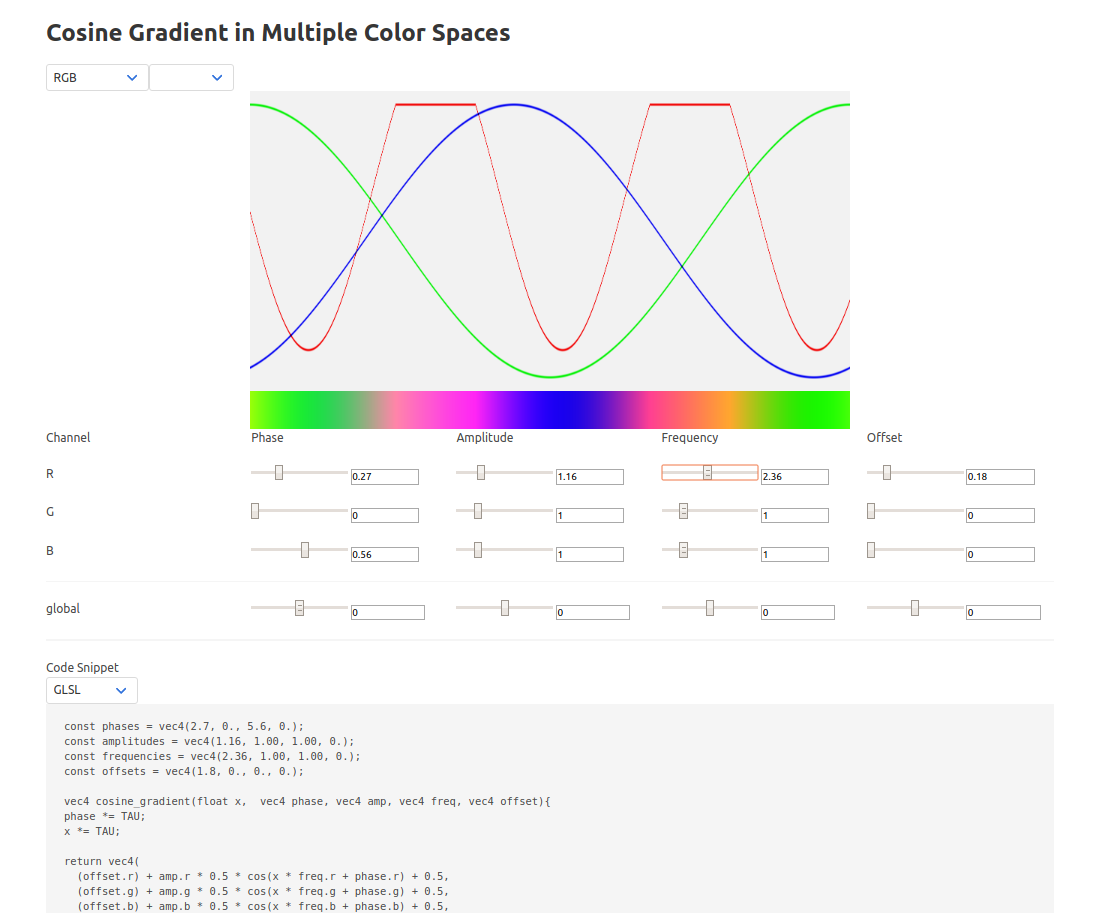$r = A_r\cos{(f_r t + p_r)} \\ g = A_g\cos{(f_g t + p_g)} \\ b = A_b\cos{(f_b t + p_b)} \\ \text{color} = (r,g,b)$